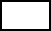Create a new printableMath Worksheets

Sample - Click above to make a new math worksheet (PDF).
 Name _____________________________Date ___________________
Functions
Complete the function table.

1.   Rule: w = 7q
 Input q 2 3 4 5 6 Output w2 * This is a pre-made sheet.Use the link at the top of the page for a printable page.
3.   Rule: k = v ÷ 6
 Input v 18 24 30 36 42 48 Output k4.   Rule: y = s + 6
 Input s 7 14 21 28 35 42 Output y5.   Rule: z = t - 13
 Input t 26 34 42 50 58 Output z6.   Rule: g = 51 - c
 Input c 1 2 3 4 5 6 7 Output g7.   Rule: h = d + 9
 Input d 3 6 9 12 15 Output h